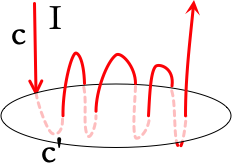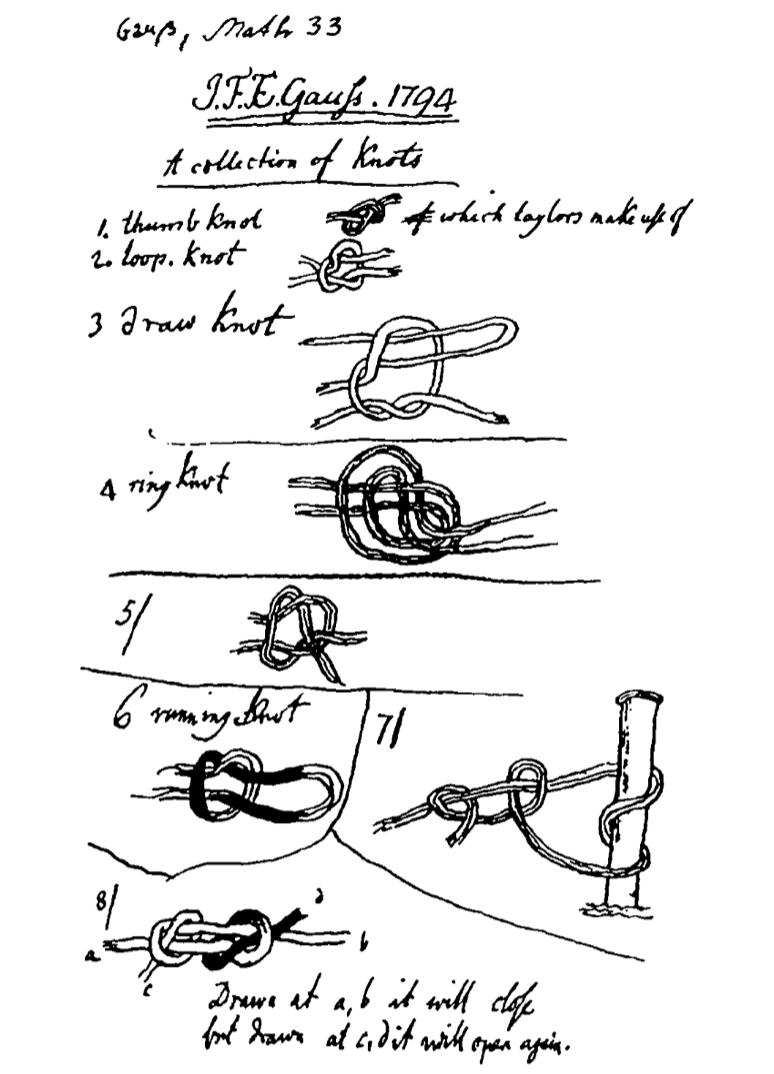# Several questions about Gauss's mathematical conception of braids

I'm trying to figure out several things about Gauss's thoughts concerning a certain four-strand braid. The reference my questions are based on is mainly Moritz Epple's excellent article "orbits of asteroids, a braid, and the first link invariant". Among the findings of this article is a new historical thesis of the role which the linking integral served in Gauss's ideas on topology, and an interpretation of it as a computational tool.

Historical references to Gauss's interest in braids

In addition, the following two excerpts from letters of two famous topologists (Betti and Mobius), refer to Gauss's interest in braids and offer interesting pieces of information:

The first citation is from an 1863 letter from Enrico Betti to Tardi:

What gave Riemann the idea of cuts was that Gauss defined them to him, talking about other matters, in a private conversation. In his writings one finds that analysis situs, that is, the consideration of quantities independently from their measure, is important; in the last years of his life he has been much concerned with a problem in analysis situs, namely: given a winding thread and knowing, at every one of its self-intersections, which part is above and which below, to find whether it can be unwind without making knots; this problem he didn't succeed in solving except in special cases...

I guess the "thread problem" which is mentioned in Betti's letter is a topological problem (on braids) in the modern sense. The second citation is taken from a letter from Mobius to Gauss on February 2, 1847:

As i heard from Wilhelm Weber, you found it nessecary, a few years ago, as an introduction or preperation for the theory of electrical or magnetic currents, to write a treatise on all possible wraps (configurations) of a thread. Is it not to be hoped that this essay will appear soon? The fulfillment of this hope will be very much appreciated by me and certainly also by many others.

The relation to Epple's interpretations

Epple's interpretation of Gauss linking integral, as a computation rather then a definition, seems to be the missing block in the reconstruction of Gauss's thoughts on topological issues, as the many indirect reports (by Betti, Mobius, Listing, etc) document. I always had a strong intuitive feeling that Gauss's introduction of the linking number cannot be just a definition, since one doesn't need an integral in order to define the linking number (one can do it simply by projecting the link into a plane and then counting negative and positive crossings).

Therefore, i believe the interpretations offered in this article must be explained more clearly, and the many misunderstandings i have regarding Epple's article prompted me to ask this question (Epple made a more accessible and thorough exposition of Gauss's fragments on topology in his book "Die Entstehung der knotentheorie", but this book is written in german so i'm unable to read it).

Some basic questions

• Epple begins his exposition of Gauss's fragment with the observation that "Gauss thought of the braid as being devided into six segments, extending from one crossing to the next". Even this basic fact is not clear to me, since the crossings devide the four strands to more than six segments.

• What are the "half-twists" mentioned in the following paragraphs?

More difficult questions

• Why did Gauss used coordinates that are based on complex integers in order to encode a braid? Epple says "That Gauss used complex integers to encode the composition of the braid might be motivated by his well known fascination with these numbers; it might, however, also have a more serious reason (see below)". Unfortunately i coudn't infer from the rest of the text the explanation for why complex numbers are important here.

• Epple suggests to connect the drawing of the braid found in Gauss's notebook with his later definition of the linking integral; according to his interpretation, the linking integral represents a computation rather then a definition - in the case of two strand braid, this analytic approach (the integral) gives rise to a linking number which equals to an integer multiple of $$\frac {{1}}{{2}}$$. If Gauss did apply a kind of linking integral for braids (how one can define linking number for a braid with more then two strands?), a question arises - are there any known definitions of a "linking number" for general braids?

• It's tempting to connect the 1847 letter from Mobius to Gauss, which mentions a treatise on all possible configurations of a "thread" (which seems to mean a "braid") as an introduction for a paper on electricity and magnetism, with this fragment, and imagine it as a detailed presentation of his thoughts on braids. However, it seems to me completely unclear how such classical physics issues are related to braids - and therefore i'd like to know if anyone is aware of connections between electromagnetism and braids and can tell something about it.

• The indirect reference in Betti's letter states that Gauss was occupied with a certain braid problem that he didn't succeed in solving except in special cases. Is there any term from modern braid theory for the topological problem described in Betti's letter?

General question

One thing that i noticed, which seems very interesting to me, is that several results or drawings which some authors refer to, don't appear in any of the 12 volumes of the published version of Gauss's werke. Just as one example, in several articles on the early history of knot theory, the authors mention a collection of 13 knots made by Gauss. So my question is: is there any website which gives access to those obscure writings of Gauss?

• Connection with electromagnetism: (see Gauss' linking number revisited for the historical context)Consider a wire $$c$$ carrying a current $$I$$, winding around a closed loop $$c'$$, as in the figure. The wire crosses the surface $$S$$ enclosed by $$c'$$ perpendicularly The integral along $$c$$ of the magnetic field $$\mathbf{B}$$ produced by the current is equal to $$\mu_0 I$$ times the linking number of the curves $$c$$ and $$c'$$. This follows directly from Ampére's law:

$$\oint_{c'}\mathbf{B}\cdot d\mathbf{l}=\int_{S} \nabla\times \mathbf{B}\cdot d\mathbf{s}=\mu_0\int_{S}\mathbf{j}\cdot d\mathbf{s}=\mu_0 I\,\text{link}(c,c').$$

If we then use the Biot-Savart law to calculate the magnetic field, $$\mathbf{B}(\mathbf{r})=\mu_0 I\oint_{c}\frac{(\mathbf{r}'-\mathbf{r})\times d\mathbf{r}'}{|\mathbf{r}'-\mathbf{r}|^3},$$ we obtain Gauss's integral expression for the linking number.

A quote by Maxwell on this result: "It was the discovery by Gauss of this very integral, expressing the work done on a magnetic pole while describing a closed curve in presence of a closed electric current, and indicating the geometrical connection between the two closed curves, that led him to lament the small progress made in the Geometry of Position since the time of Leibnitz, Euler and Vandermonde. We have now, however, some progress to report chiefly due to Riemann, Helmholtz and Listing." [see arXiv:0703086.]

The general question:
Gauss's drawings of knots are in his 1794 notebook, which I have not found online. A copy of one page is in History of Science and Knots, see screenshot.Did Gauss write in English?

The book from which I copied this page with knots suggests that, indeed, the 17-year old Gauss wrote it himself in English:

In 1794 Gauss prepared sketches of thirteen knots, with English names, perhaps copied from an English book of seafarers' knots; this note, together with other papers bearing sketches of knots, was found amongst his papers after his death in 1855.

Below is a signature from Gauss in 1841, almost half a century after these notes, but the handwriting looks quite similar (compare the G and ß in his last name).• This is a copy from Gauss notebook? Gauss wrote his notebook in English?? Feb 6, 2021 at 1:49
• Gauss wrote his math diaries in Latin. Feb 6, 2021 at 4:48